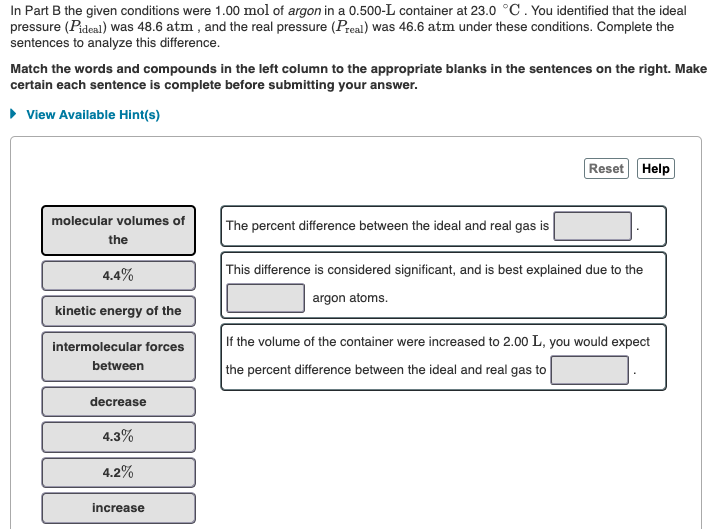Home / Expert Answers / Chemistry / in-part-b-the-given-conditions-were-1-00-mathrm-mol-of-argon-in-a-0-500-mathrm-l-pa897

# (Solved): In Part B the given conditions were $$1.00 \mathrm{~mol}$$ of argon in a $$0.500-\mathrm{L}$$ ...In Part B the given conditions were $$1.00 \mathrm{~mol}$$ of argon in a $$0.500-\mathrm{L}$$ container at $$23.0{ }^{\circ} \mathrm{C}$$. You identified that the ideal pressure ( $$\left.P_{\text {ideal }}\right)$$ was $$48.6 \mathrm{~atm}$$, and the real pressure $$\left(P_{\text {real }}\right)$$ was $$46.6$$ atm under these conditions. Complete the sentences to analyze this difference. Match the words and compounds in the left column to the appropriate blanks in the sentences on the right. Make certain each sentence is complete before submitting your answer. The percent difference between the ideal and real gas is This difference is considered significant, and is best explained due to the argon atoms. If the volume of the container were increased to $$2.00 \mathrm{~L}$$, you would expect the percent difference between the ideal and real gas to

We have an Answer from Expert